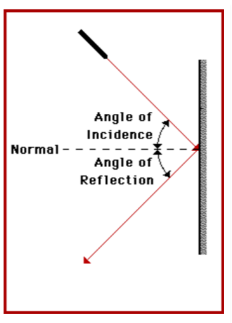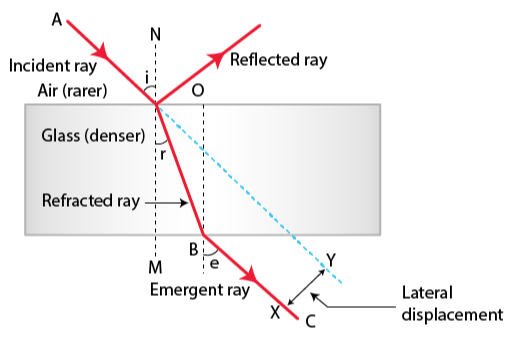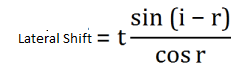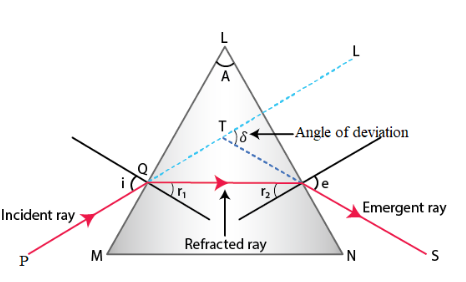Checkout NEET 2022 Question Paper Analysis : Checkout NEET 2022 Question Paper Analysis :

# Geometrical Optics Formulae for NEET

1. Laws of Reflection of light• The incident ray, the refracted ray, and normal lie on the same plane
• Snells law

sin i/sin r = constant

2. Relative refractive index 1n2 = v1/v2

v1 = velocity of light in first medium

v2 = velocity of light in second medium

3. Absolute Refractive indexn = c/v

c= velocity of light in air

v = velocity of light in given medium

4. Lateral Shift5. Normal shift on a single surfaceNormal shift Ns= t[1-1/n]
6. Relation between refractive index and critical anglen = 1/sin C
7. Refraction through a prismRefractive index of the prism n = [sin(A+δ/2)]/sin A/2

8. Deviation produced by a thin filmd = (n-1) A

(n-1) is called the refractivity of the material

9. Angular dispersion between two coloursAngular dispersion = (nv-nr)A

nv, nr is the refractive index of violet light and red light

10. Dispersive powerω = nv– nr/n-1
11. Lens makers formula for thin lenses1/f = (n-1) { 1/R1 – 1/R2}
12. Power of lensP = 1/f
13. Equivalent focal length of combination of two thin lenses1/f = 1/f1 + 1/f2

Searched articles: